# Time Series Analysis — An Intro to Predicting Crypto Token Performance

If only you could know how well your crypto will do in the future… Finally your high school math comes in handy, as you can get an idea by punching token data into formulas and models. Here is how.

May 27, 2019
|

## The Purpose of Analysing a Time Series Dataset

With the burgeoning data deluge in various sectors, the world has been eager to glean valuable insights from data analytics. Here we introduce some basic tools for analysing time series data, which is particularly prevalent in financial markets.

A time series refers to a time-ordered set of observations on the values that a variable takes, and such data is usually collected at regular time intervals. As for financial and economic data, typical examples include closing prices of stocks (available weekdays), consumer price indices (released monthly or quarterly), and government budgets (announced annually).

Statisticians and economists have developed complicated theories and tools for analysing time series data. The goal of time series analysis is threefold: describing data patterns, explaining phenomena, and making predictions. This article aims to provide some intuition based on understanding the basic concepts of the analysis on time series data.

## Step 1: The Components of a Time Series

#### Time plots: The base of it all

Before performing any fancy statistical techniques, the first and foremost thing we should always do is to make a time plot with the data. A time plot is simply a graph with observations plotted against time. We can first get an intuitive feel of the patterns and features of the data, and then move on to more detailed descriptive or modelling techniques for further investigation. With the help of time plots, we can know the three main components of time series data, namely, trend, seasonality, and cyclicality.

#### Trends: Where are things heading?

trend is a slow and long-term evolution in the data. For example, housing prices tend to have a long-term upward trend over tens of years, and this can be caused by long-term growth of population and economy. Also, despite short-term fluctuations, the S&P 500 Index has had an apparent uptrend for the last 10 years. A trend can be linear or nonlinear. A linear trend implies that the variable grows at a constant speed over time, while the growth rate of a non-linear trend is changing over time.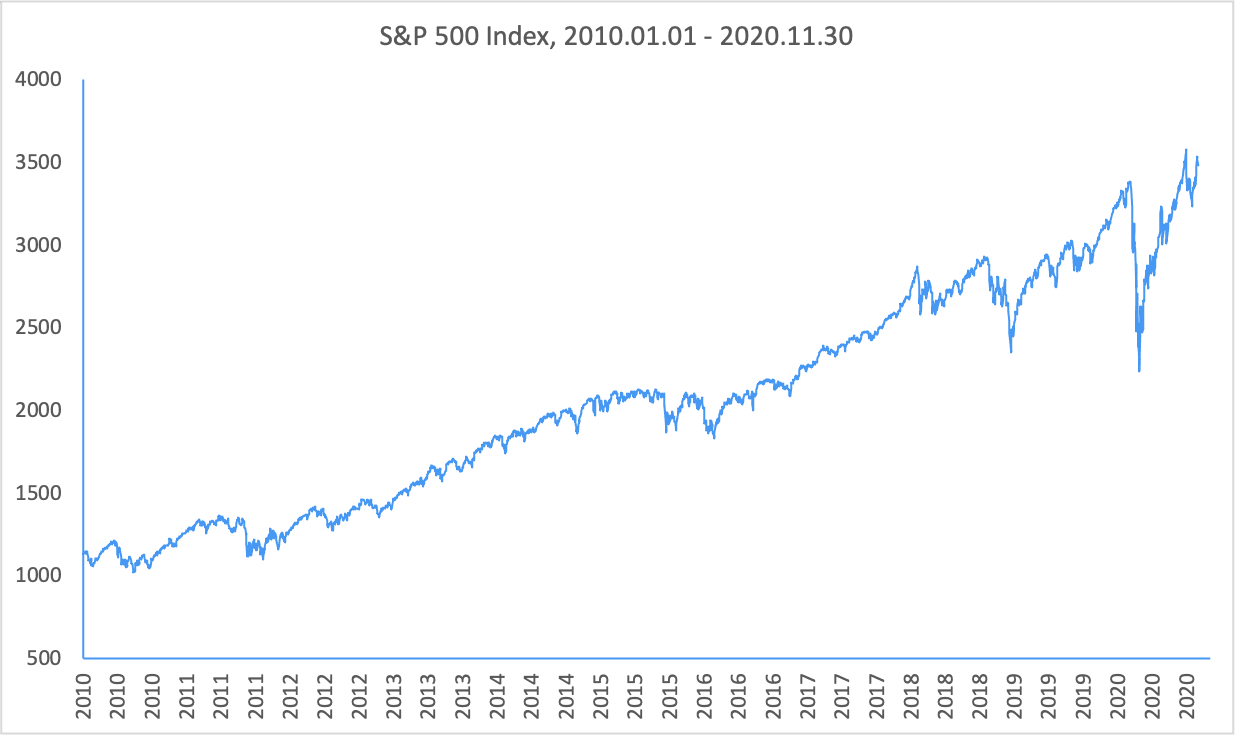#### Seasonality: Things are changing

A seasonal pattern exists when a series is impacted by seasonal factors such as the month of the year or the day of the week. In other words, a seasonal pattern is one that repeats itself in regular time intervals. For instance, the raw figures of consumption expenditures are typically higher in the fourth quarter thanks to extra spending during the Thanksgiving and Christmas holidays. As seen in the graph, the personal consumption expenditures in the US display an uptrend with apparent seasonality.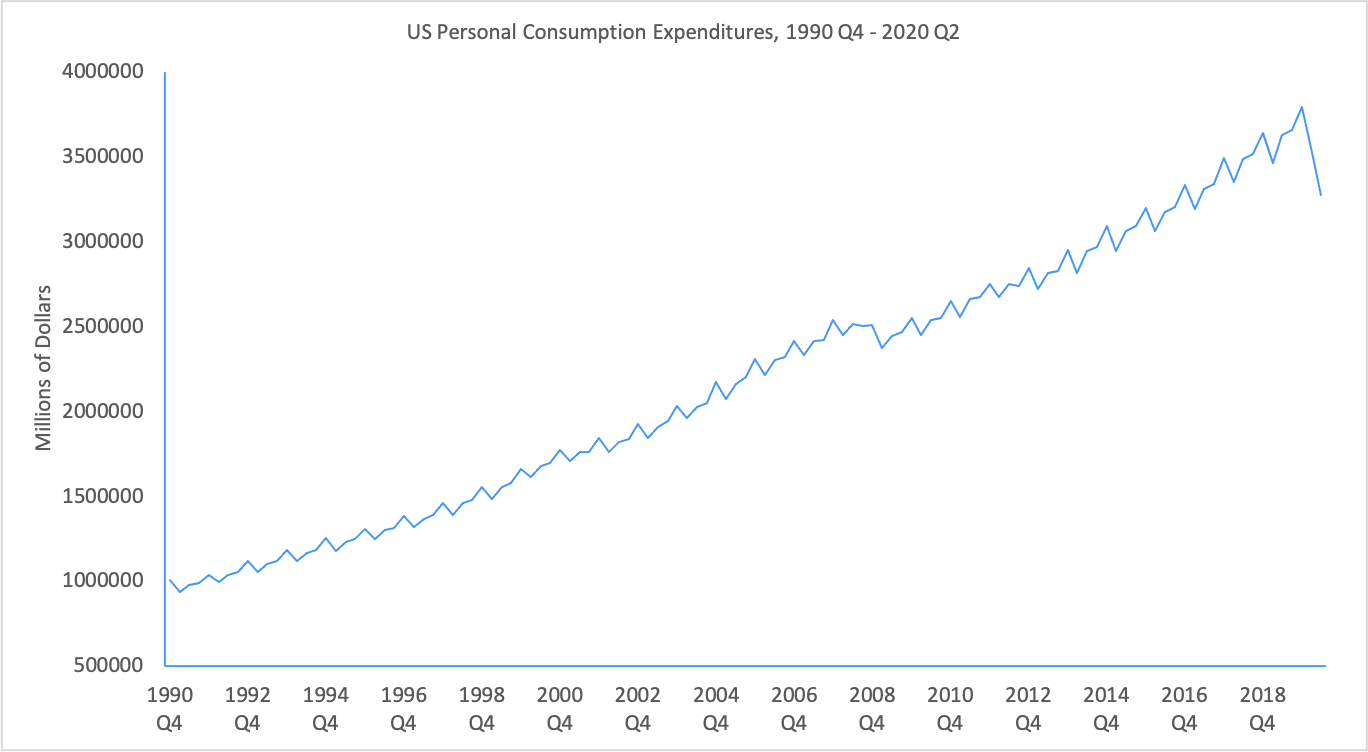#### Cyclicality: Things repeat

We usually perceive a cycle as a routine up-and-down pattern in the data, but the meaning of cyclicality in time series analysis has a much broader coverage than that. Cyclicality here includes any dynamics and persistence other than trends and seasonality in the data. Such dynamics and persistence represent connections between the past and the present, and the future and the present. Cycles can be found in many time series, as data in different time points are usually correlated to some extent. The graph below is a time plot of the new one-family houses sold in the US. We can see persistent and irregular upward and downward movement in sales over years, a dynamic that indicates strong cyclical behaviour.

## Step 2: Calculating a Regression Analysis and Correlation

#### Regression Analysis

Here we illustrate the idea of regression analysis, which is essential for understanding the modelling of time series.

Regression analysis helps identify how two or more variables are related. For example, we can utilise regression to explore whether Ethereum returns are related to Bitcoin returns.

The most basic form of regression is linear regression with only one independent variable. This form is based on a linear function:The variable y is the dependent variable, and the variable x is the independent variable. The graph of such an equation is a straight line in an x-y plot. The constant a is the y-intercept of the line while the constant b is the slope measuring the steepness of the line.

Linear regression is in fact a practice that fits a straight line on data points. The purpose is to find a linear function of x that gives the best approximation or forecast of y. What defines a ‘best’ approximation? In most cases, the strategy is to minimise the total of the squared vertical distance between the data points and the fitted line. This famous estimation strategy is called least squares.

Who is the refresher on high school math feeling so far? Now let us try a linear regression with the data of daily Ethereum return and daily Bitcoin return in 2019. We use daily Ethereum return as the dependent variable and daily Bitcoin return as the independent variable.

In the figure below, we graphically illustrate the results of regressing Ethereum return on Bitcoin return. The data points on each trading day in 2019 are all plotted, and a positive-sloped fitted line is obtained from running the linear regression. The slope tells us how the changes in the two variables are related. We can see that the slope is 0.711, and it implies that, on average, for every one percentage point increase in daily Bitcoin return, daily Ethereum return increases by 0.711 percentage point.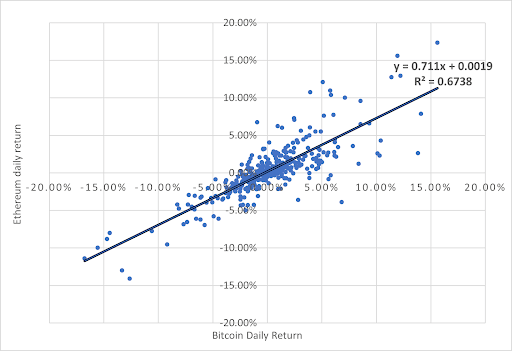Now we want to find out whether the straight line is a good predictor or not.

R-squared, usually referred to as R2, is a common measure of goodness of ﬁt of regression models. It refers to the proportion of the variance of the dependent variable that can be explained by the independent variables in a regression model.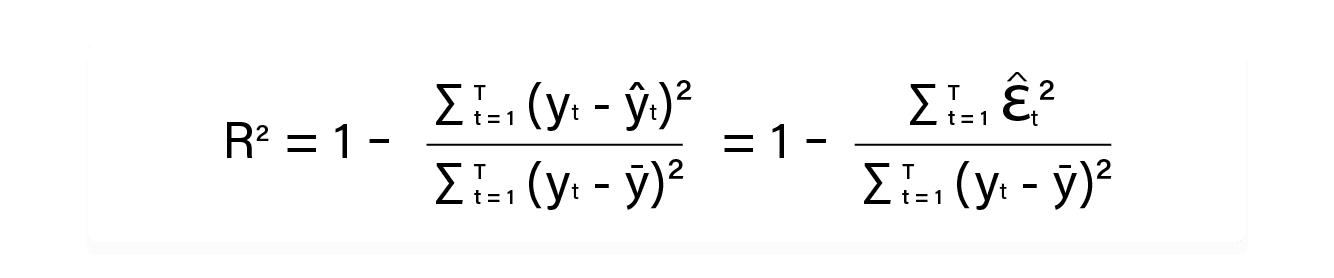As seen from the equation: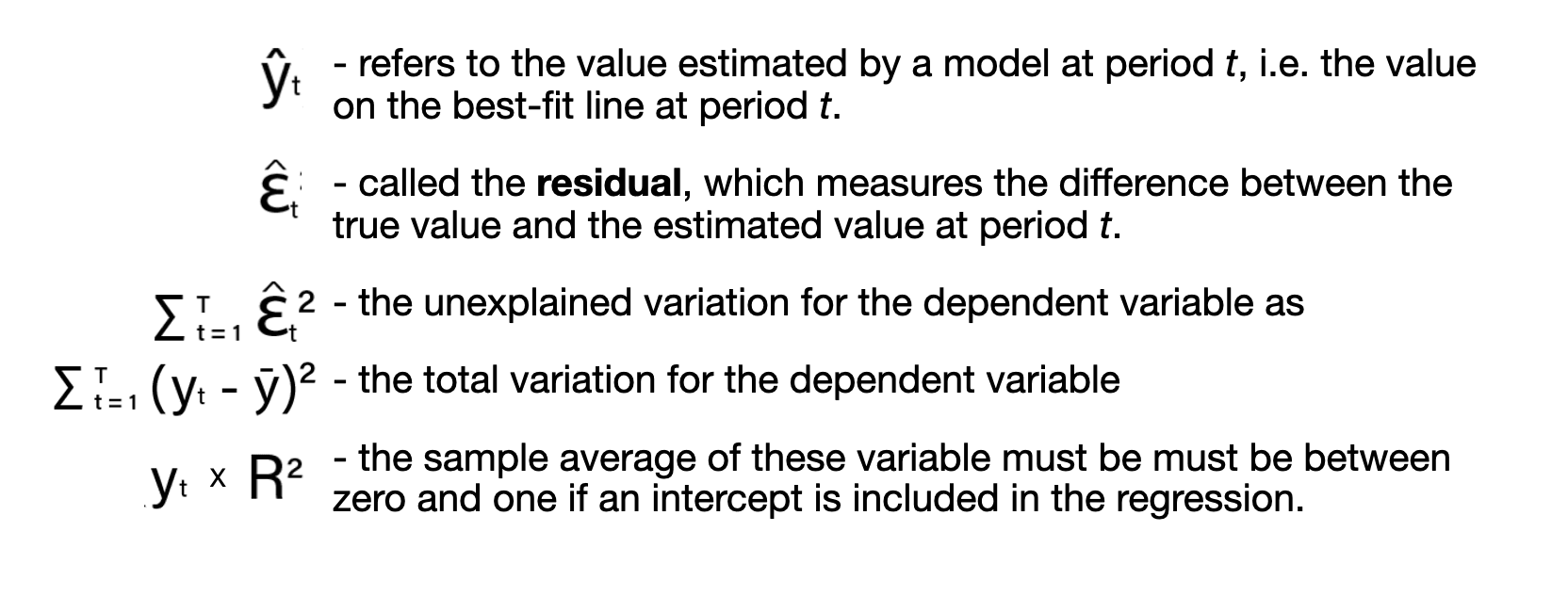You got that?

Then, the takeaway is clear: the closer the value of R2 to 1, the better the approximation or predictability provided by the model, and vice versa. In our example, R2 equals 0.6738 and it means that using our best-fit line, around 67.4% of the variation in daily Ethereum returns can be explained by the variation in daily Bitcoin returns. This figure shows a fairly strong goodness of fit.

#### Correlation

Additionally, we can use the correlation coefficient, r, to measure the strength and direction of correlation, i.e. the linear association between the dependent and the independent variable.This coefficient always ranges between -1 and +1. A positive r implies a positive correlation, while a negative implies a negative correlation. The closer the value of r to -1, the stronger is the negative correlation; the closer the value of r to +1, the stronger is the positive correlation. As for our example, r is equal to 0.821, which indicates a strong and positive correlation between daily Ethereum returns and daily Bitcoin returns.

The simple linear regression with one single independent variable can be further extended to a multiple linear regression model with multiple independent variables. Also, we need to keep in mind that regression results and correlation coefficients only provide evidence for correlation between variables, and this does not imply causation.

## Step 3 (Finally): Predicting Where Things Go by Modelling Trends

We have talked about the three main components of time series data and the idea of regression, and we now explore some basic models that can be adopted to measure the time series components quantitatively.

There are two types of trends: deterministic trends and stochastic trends. The former one implies that the future is perfectly predictable whereas the latter indicates that the future is only partly dependent on the past and exact predictions are impossible. Here we focus on modelling the simpler deterministic trends.

#### Linear trends

Let us start with modelling a linear trend. It is simply a linear form of time:Now suppose we have the price data of a token for T time periods, and we want to examine whether the price level follows a linear trend. Here, yt is the token price we are trying to model. TIME is an indicator variable constructed for specifying the time order of the periods. It equals 1 for the first period, i.e. TIME=1 when t=1, and 2 for the second period, and so on. The yand TIMEvariables indicate their values at their corresponding time periods t=1,2,…,T. βis the intercept and it specifies the value of yt at t=0. βis the slope; it is positive for an increasing trend and negative for a decreasing trend. We can interpret it this way: as when the time passes by one period, yt grows by an amount of β1. εis the error term covering anything not captured by the trend component. In practice, we can simply estimate the values of β0 and βwith least squares regression.

We all know that trends may not be linear. For example, a company’s stock price may grow faster and faster with accelerating growth in sales. In other words, the price can increase at an increasing rate instead of a constant rate with respect to time. We also have models catering for non-linearity.

quadratic trend model is a case in point. As illustrated by the name, it is expressed as a quadratic function:For instance, as shown in the following graph, if both 1 and 2 are larger than zero, the value grows at an increasing rate with respect to time: the rise of the value in every period increases as time goes on.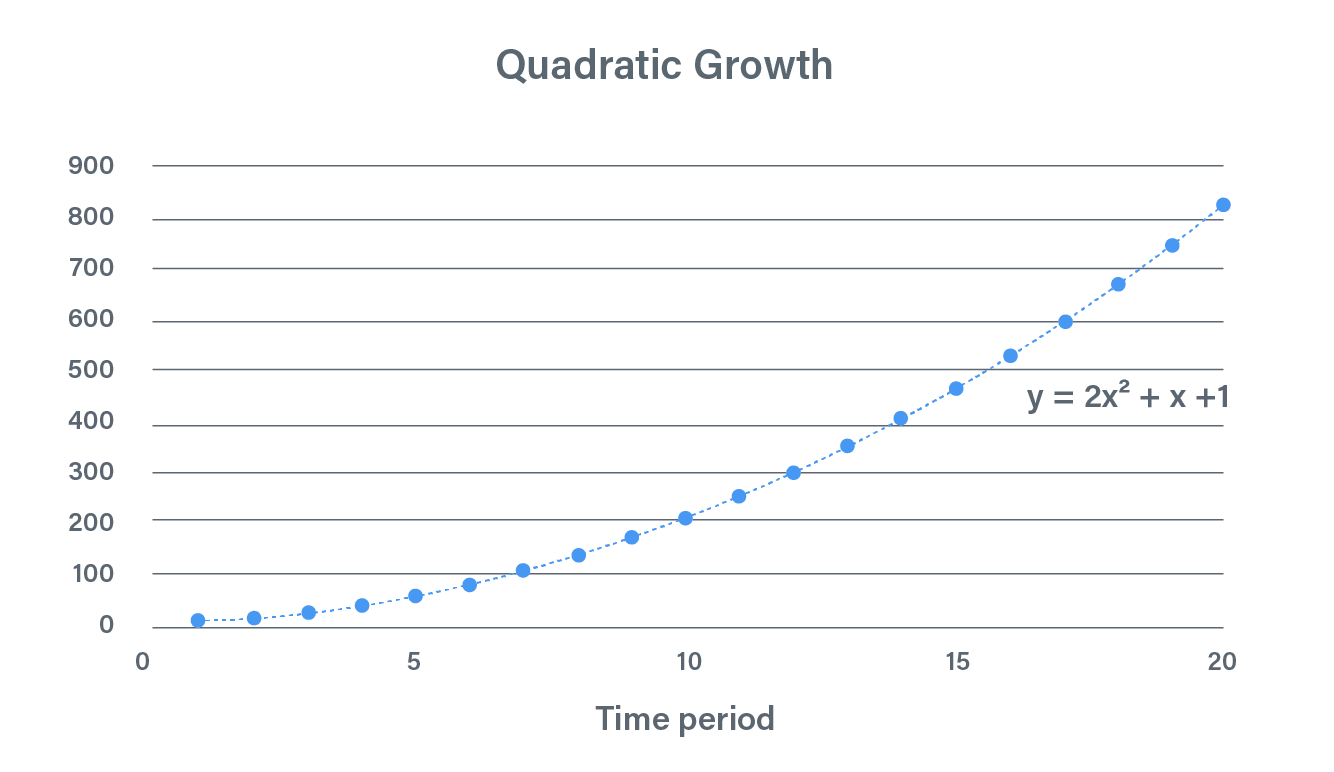#### Exponential trends

Another prominent type of trend is exponential trend. Such trends can be easily found in financial and economic time series as many of them grow by a roughly constant percentage growth rate per year. Say the general price levels in a country may grow at around 2% every year. The meaning of growth rate here is different from the rate we mentioned in the quadratic trend model. It is constant in the sense that its own value grows at the same percentage for every period. However, the rise of the value in every period actually increases in quantity as time goes on, and this is the increasing rate we mentioned before.

The level form of an exponential trend is expressed as what follows and βis the growth rate:We can see that it is a nonlinear function of time, but after taking natural logarithm on both sides, we can obtain its linear form:We can then estimate the growth rate βwith simple linear regression. The following two graphs illustrate an exponential growth trend with a constant 20% growth rate per period and also its transformed shape. We can observe that after taking natural logarithm on the exponential trend, we can have a simple linear trend for estimation.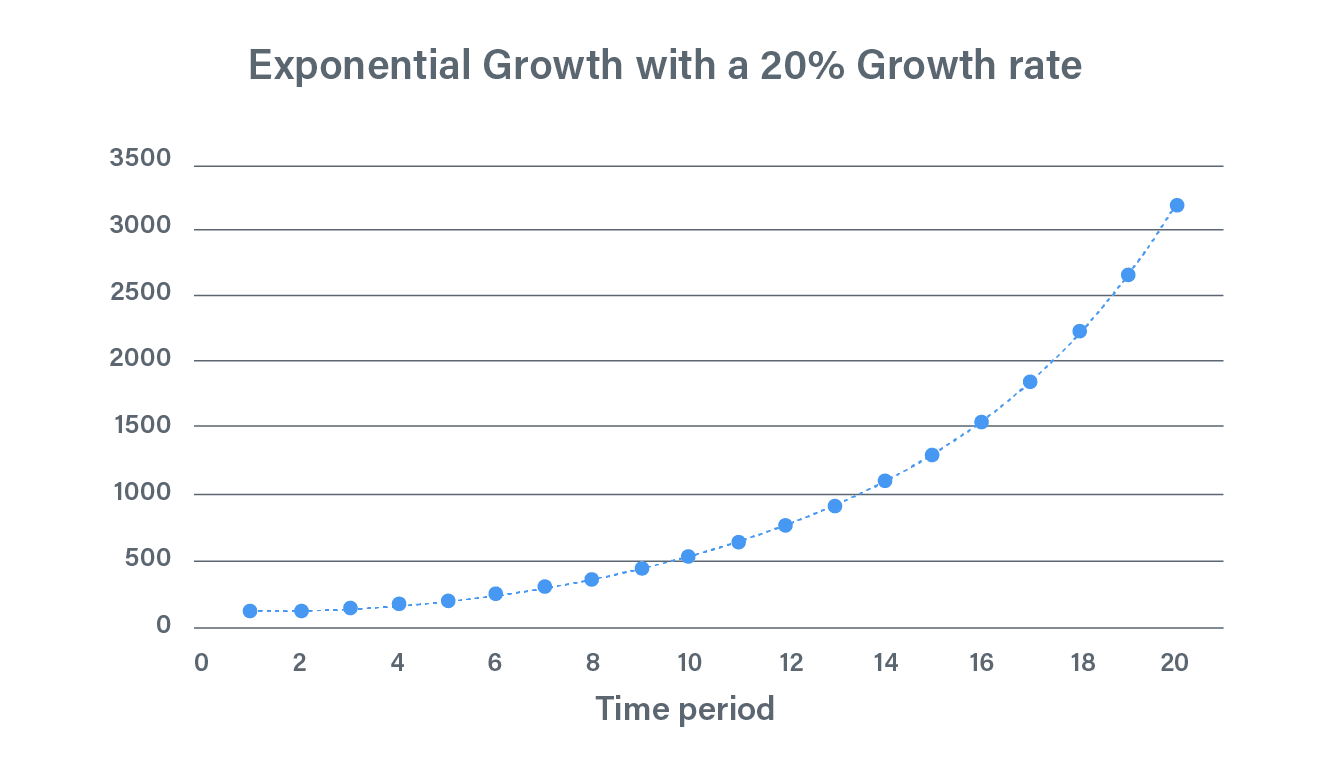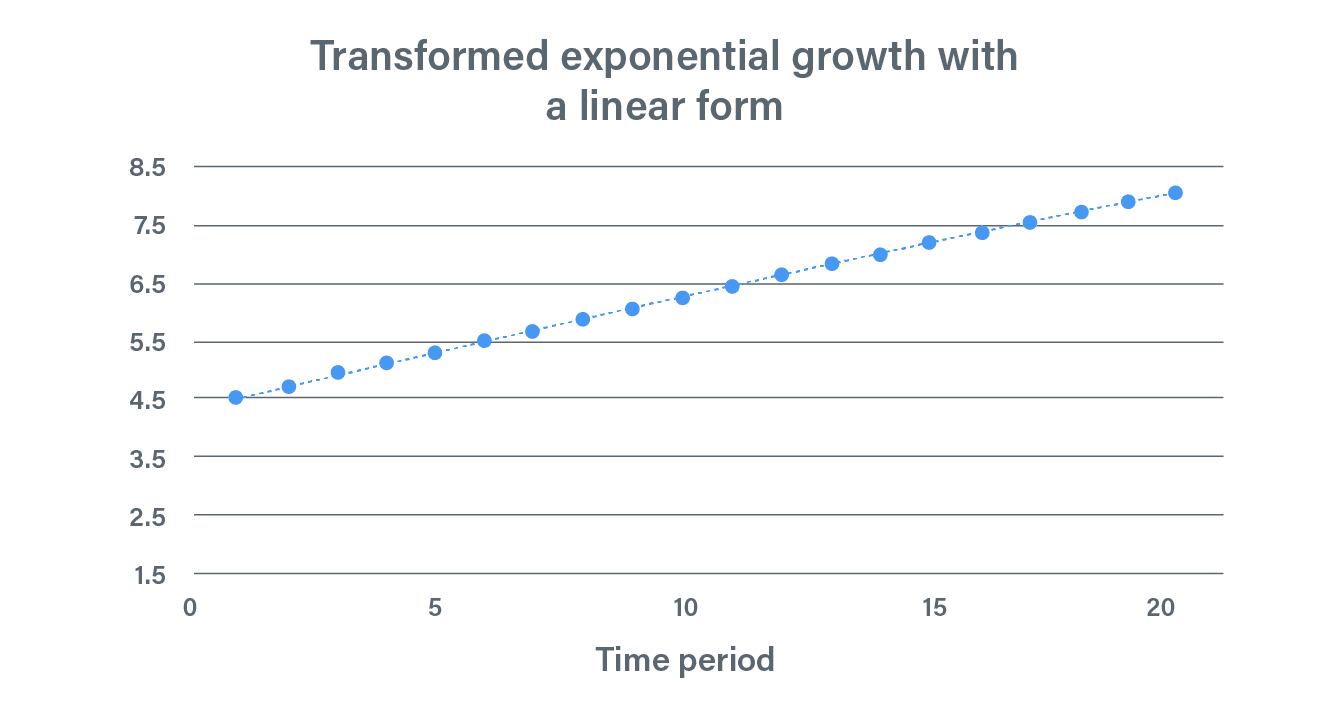## Step 4: Adding Another Factor by Modelling Seasonality

As for modelling repetitive calendar patterns, we have two types of models: additive seasonality and multiplicative seasonality.

For additive seasonality, the impact of seasonality is a constant quantity over time. On top of the trend component as discussed in the former section, we can add a seasonal factor to capture the seasonal factor.Assume that in a city, the consumer spending increases by around 100 million dollars in December every year. Then we can use additive seasonality to capture the impact.

#### Multiplicative seasonality

However, such additive seasonality may not be readily found in reality as the seasonal impact exists more as a percentage increase or decrease on the normal values. So, a more common example is that the consumer spending rises by 10% in December as compared to the annual mean level. And the following is the expression for modelling multiplicative seasonality:In short, we should use the additive one when the seasonal impact is roughly constant over time, and we should use the multiplicative one when the seasonal impact rises over time. After making seasonal adjustments, we can observe the variations in data caused by factors other than regular seasonal effects.

## Step 5: Going Round and Round Through Modelling Cycles

#### Stationarity

Now we go over some models related to cyclicality, the more complex component in time series data. We first need to learn about a key concept called stationarity. A stationary time series is one whose key properties are stable over time. These properties include mean, variance, and autocovariance of the time series.

To put it simply, we hope that there is a set of rules governing both the future and past of a series, so that we can make predictions based on these rules. An autoregressive (AR) model can model cycles by explicitly regressing a variable against the lagged values of itself.

AR(1) only involves the value in the previous period as the independent variable:The current value is linearly related to the past value, plus a random shock εt. The series here needs to be stationary and the absolute value of φ should be smaller than 1. AR(q) is a multivariable regression model with independent variables lagged up to q periods.

#### Moving average models

Instead of using past values of the dependent variable in a regression, a moving average (MA) model predicts the dependent variable as a function of current and past random shocks. In an MA(1) model, the current value is a function of current shock and the shock of the previous period:The assumptions and mathematics behind the ARMA models are rather involved, and if you are interested in the practical applications of these models, take a look at the references listed at the end of this article.

### Step 6: What’s a Good Fit? Tools for Model Selection

After getting into the details of how the models work, we certainly want to know how well they fit the data. We have mentioned R2, which is definitely the most basic indicator for modeling fitting. Here are some other important indicators and tools we usually examine for model selection:

#### The Akaike information criterion (AIC) and Schwarz information criterion (SIC)AIC and SIC are constructed in a similar way as R2. e2k∕T and Tk∕T are factors added for punishing the over-use of explanatory variables, which may cause the problem of overfittingk is the number of explanatory variables used in the regression. We can observe that the values of AIC and SIC increase with k. The smaller the values of AIC and SIC, the better the explanatory power of the model. SIC has a harsher penalty for the number of explanatory variables involved.

#### The Durbin-Watson Statistic

If our model has exhausted all the major factors driving the time series, we should not observe any forecastable patterns in the error terms. The following illustrates a common case in which the error terms are forecastable: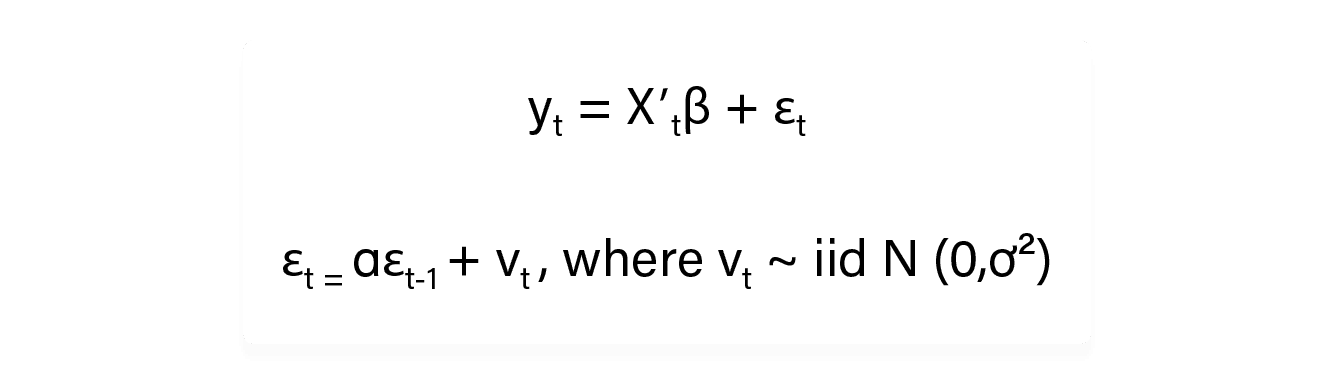Despite the symbols involved, the main idea here is not hard to grasp. The first line is the regression model. And the hypothesis we are going to test lies in the second equation, which means that εt, an error term at any period t, is correlated with εt-1, the error term in the previous period t-1. It is usually called serial correlation. The hypothesis of interest is that α=0, which means that serial correlation does not exist among the error terms. This implies that our model does not neglect any prominent patterns in the series. The Durbin-Watson Statistic is designed for testing this hypothesis with residuals resulting from the regressions:The values of DW range between 0 and 4. If DW is around 2, we have the ideal case of zero serial correlation. If DW is much larger than 2, the error terms are negatively correlated; if DW is much less than 2, the error terms are positively correlated. If serial correlation is identified in the residuals, we probably need to add factors into the original model so as to capture the remaining dynamics in the data.

## What’s Next?

We have discussed some key concepts about modelling time series data. You can start with some time series data you are interested in and play around with some simple methods and models we have mentioned. Most of these methods can be easily implemented in some common statistical software and programming languages.

Do keep in mind that what these models are doing is gleaning knowledge about the future by looking at the past. They extract and highlight trends, but much of the future is unpredictable – like Covid-19 was. We certainly didn’t see that coming.

This article only serves as a very brief introduction. You probably need to know more about the statistical concepts for a comprehensive understanding of time series analysis, and the links in the article and the reference list below provides more essential knowledge. Also take a look at our article series on valuating crypto assets to become a pro at understanding what moves the crypto market.

## References

1. Diebold, F.X. (2019). Econometric Data Science: A Predictive Modeling Approach, Department of Economics, University of Pennsylvania. http://www.ssc.upenn.edu/~fdiebold/Textbooks.html.
2. Hyndman, R.J., & Athanasopoulos, G. (2018). Forecasting: Principles and Practice. OTexts: Melbourne, Australia. https://otexts.com/fpp2/.
3. OpenStax College. (2013). Introductory Statistics. OpenStax. https://openstax.org/details/books/introductory-statistics.
4. The Pennsylvania State University. (2020). Applied Time Series Analysis. https://online.stat.psu.edu/stat510/.

#### Tags

crypto

DYOR

Finance

financial models

investment

time series analysis